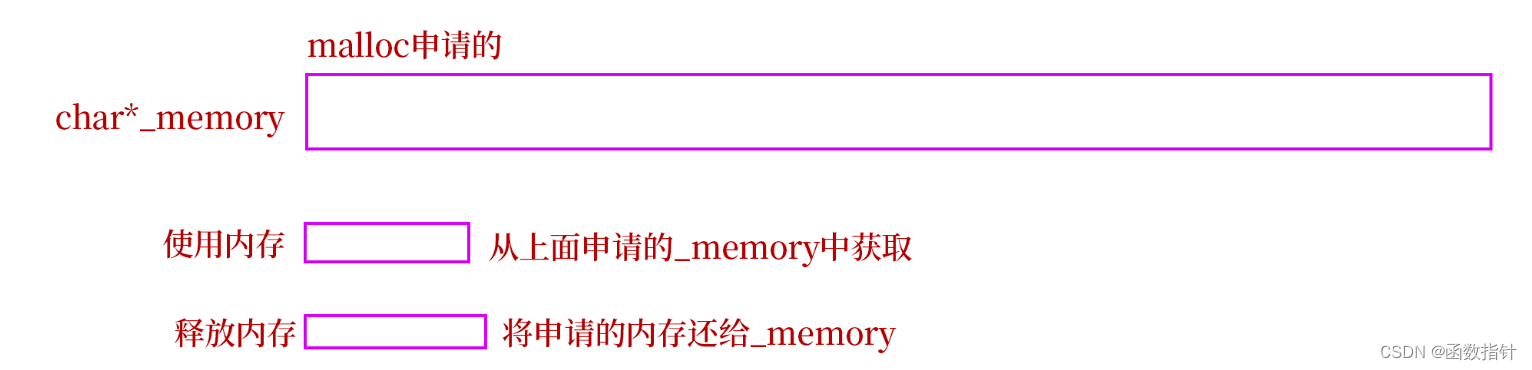# C++--简单实现定长内存池

1.什么是定长内存池

在C/C++中，动态申请内存都是通过malloc来申请的，但是实际上不是是直接从堆上直接申请的内存，而是通过malloc动态申请一大块内存，malloc就相当于一块内存池，然后分给程序使用，如果申请的内存使用完或者不够需要申请的内存超过当前剩余的内存的时候，再去向操作系统申请内存。

内存池主要解决的是在动态申请内存的时候，解决效率的问题，当然，还可以解决一些内存碎片化问题。

定长内存池就是通过malloc向操作系统申请一大块内存，然后分给需要使用的程序使用。用完之后还给内存池的行为。但是，功能单一，只能解决定长的内存需求，另外占着内存没有释放。``````#pragma once
#include <iostream>
#include <vector>
#include <time.h>

using std::cout;
using std::cin;
using std::endl;

namespace sss
{
template<class T>
class Objectpool
{
public:
T* New()//开辟大小
{
T* obj = nullptr;
//内存重复利用
if (_freeleft)
{
void* next = *(void**)_freeleft;
obj = (T*)_freeleft;
_freeleft = next;
}
//开辟空间
else if (_reminBytes <sizeof(T))
{
_reminBytes = 128 * 1024;
_memory = (char*)malloc(_reminBytes);
if (_memory == nullptr)//判断是否为空
{
}
}

obj = (T*)_memory;
size_t objSize = sizeof(T) < sizeof(void*)
? sizeof(void*) : sizeof(T);
//保证在32位或64位下性能正常使用，因为一个指针最小为4字节

_memory += sizeof(T);//往后加内存
_reminBytes -= sizeof(T);//剩余内存
//定位new;

new(obj)T;//调用T的构造函数

return obj;

}

void Delete(T* obj)//释放内存
{
obj->~T();
/*if (_freeleft == nullptr)
{
_freeleft = obj;
obj = nullptr;
}*/
//头插
*(void**)obj =_freeleft;//32位64位都可以运行
_freeleft = obj;
}
private:
char* _memory;//开辟的大小
size_t _reminBytes=0;//剩余的大小
void* _freeleft;//
};
}``````